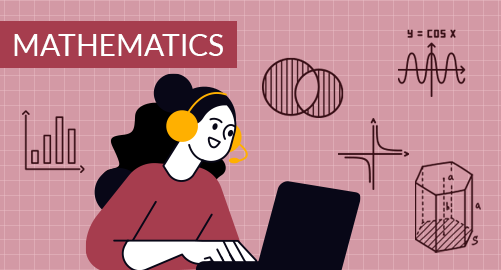#### Need Help?

Get in touch with usMathematics

Grade 8 Mathematics covers Real numbers, Linear equations, Functions, Statistics, Congruence and Similarity, Pythagorean Theorem and Perimeter, Area, Solve Problems Involving Surface Area and Volume

## What you'll learn

• 1

Real Numbers: Rational Numbers as Decimals, Understand Irrational Numbers, Compare and order Real Numbers, Evaluate Square roots and Cube Roots, Definition of an exponent& Properties of Integer Exponents, Solve Equations Using Square Roots and Cube Roots.

• 2

Linear equations: Combining Like terms to solve equations, Solving Equations with Variables on both sides, Equations with No solution or infinitely many solutions, Comparing and Connecting Proportional Relationship and Slope, Analyzing equation of the form y = mx + b, Estimate Solutions By Inspection, Solving System of Linear Equations by Graphing, Substitution and Elimination.

• 3

Functions: Understand Relations and Functions, Connect Representation of Functions, Compare Linear and Non-Linear Functions, Construction of Functions to Model Linear Relationships, Intervals of Increase and Decrease, Sketching of Function from the verbal Descriptions.

• 4

Statistics: Construction and Interpretation of Scatter Plots, Analyzing Linear Associations, Use of Linear models in Making Predictions, Interpretation of Two-Way Frequency Table and Relative Frequency Table.

• 5

Congruence and Similarity: Translations, Reflections, Rotations, Compose Transformations, Understanding Congruent Figures, Dilations, Understanding Similar Figures, Lines, Angles and Transversals, Interior and Exterior Angles of Triangles, Angle-Angle Triangle Similarity.

• 6

Pythagorean Theorem and Perimeter, Area: Understanding Pythagoras Theorem and Converse of Pythagoras Theorem, Application of Pythagoras Theorem, Finding Distance Between Two Points in Coordinate Plane, Area of Parallelograms, Triangles, and Trapezoids, Circumference and Area of Circles, Area of irregular figures.

• 7

Solve Problems Involving Surface Area and Volume: Finding Surface Area of Three-Dimensional Figures, Finding Volume of Cylinders, Cones, Spheres.

## Skills you'll learnIdentify an appropriate mathematical rule or procedure based on the classification of a given expressionExplain how an approximated value relates to the actual valueIdentify mathematical information from graphical, numerical, analytical, and/or verbal representationsDescribe the relationships among different. representations of functions and their derivativesExplain the meaning of mathematical solutions in contextIdentify an appropriate mathematical definition, theorem, or test to applyUse precise mathematical language and appropriate units of measure and symbols